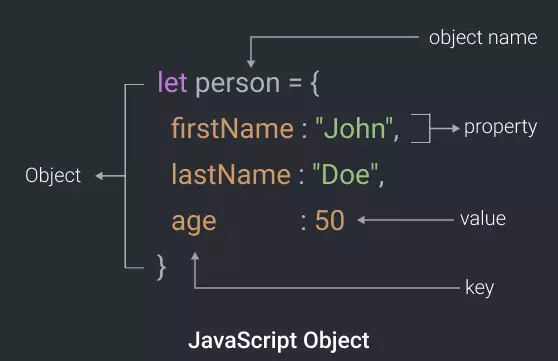# How to Get Subset of JS Object Properties

JavaScript objects are powerful data structures that allow you to easily store different types of data in a compact manner. Sometimes you have a large JS object with lots of data but you only need to use a small part of it. In such cases, it is advisable to take a subset of the JS object and then continue working with it. Otherwise, it can slow down your code. In this article, we will learn how to get subset of JS object properties.

## How to Get Subset of JS Object Properties

Let us say you have the following JS object.

`obj = { a: 1, b: 2, c: 3  };`

Let us say you want to retrieve the following subset of above JS object.

`object = { b: 2, c: 3  };`

There are many ways to do this but we will look at a couple of simple ways.

## 1. Using Destructuring

Destructuring Assignment allows you to easily unpack values from arrays, or properties from JS objects. You can easily extract the required subset using the following command. Here is an example where we get subset object containing properties ‘b’ and ‘c’ in object test.

```subset = (({ b, c }) => ({ b, c }))(object);
console.log(subset); // { b: 2, c: 3 }```

You can also use another way to destructure the above JS object. In this case, you need to prefix the subset object with ‘…’ and assign it to the original object, as a part of another temporary object.

```{a,...subset}=test;
console.log(subset);// {b:2, c:3}```

The syntax of destructuring is a little unusual and we suggest that you refer to the link we have provided above, to get a detailed idea about destructuring assignment.

## 2. Using Lodash/Underscore

Underscore is a popular JS library that provides a simple pick() function to extract a subset of JS object. You can also use Lodash, which is similar to Underscore.

`var subset = _.pick(test, ['b', 'c']);`

In this article, we have learnt how to get subset of JS object properties.